# Plotting data

The Python Control Toolbox contains a number of functions for plotting input/output responses in the time and frequency domain, root locus diagrams, and other standard charts used in control system analysis, for example:

```bode_plot(sys)
nyquist_plot([sys1, sys2])
```

While plotting functions can be called directly, the standard pattern used in the toolbox is to provide a function that performs the basic computation or analysis (e.g., computation of the time or frequency response) and returns and object representing the output data. A separate plotting function, typically ending in _plot is then used to plot the data, resulting in the following standard pattern:

```response = nyquist_response([sys1, sys2])
count = response.count          # number of encirclements of -1
lines = nyquist_plot(response)  # Nyquist plot
```

The returned value lines provides access to the individual lines in the generated plot, allowing various aspects of the plot to be modified to suit specific needs.

The plotting function is also available via the plot() method of the analysis object, allowing the following type of calls:

```step_response(sys).plot()
frequency_response(sys).plot()
nyquist_response(sys).plot()
rootlocus_response(sys).plot()     # implementation pending
```

The remainder of this chapter provides additional documentation on how these response and plotting functions can be customized.

## Time response data

Input/output time responses are produced one of several python-control functions: `forced_response()`, `impulse_response()`, `initial_response()`, `input_output_response()`, `step_response()`. Each of these return a `TimeResponseData` object, which contains the time, input, state, and output vectors associated with the simulation. Time response data can be plotted with the `time_response_plot()` function, which is also available as the `plot()` method. For example, the step response for a two-input, two-output can be plotted using the commands:

```sys_mimo = ct.tf2ss(
[[, [0.1]], [[0.2], ]],
[[[1, 0.6, 1], [1, 1, 1]], [[1, 0.4, 1], [1, 2, 1]]], name="sys_mimo")
response = step_response(sys)
response.plot()
```

which produces the following plot: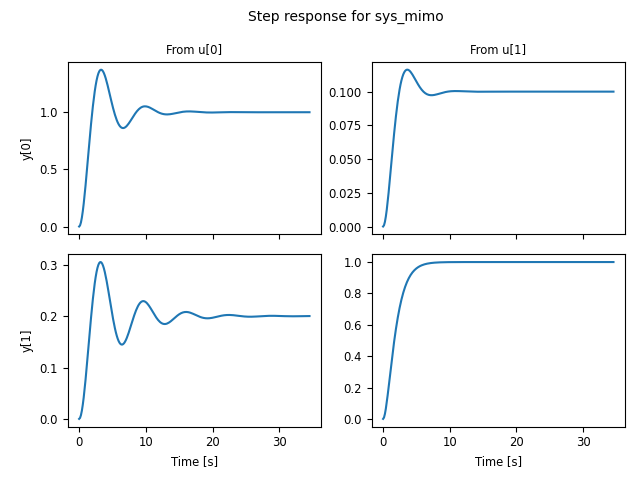The `TimeResponseData` object can also be used to access the data from the simulation:

```time, outputs, inputs = response.time, response.outputs, response.inputs
fig, axs = plt.subplots(2, 2)
for i in range(2):
for j in range(2):
axs[i, j].plot(time, outputs[i, j])
```

A number of options are available in the plot method to customize the appearance of input output data. For data produced by the `impulse_response()` and `step_response()` commands, the inputs are not shown. This behavior can be changed using the plot_inputs keyword. It is also possible to combine multiple lines onto a single graph, using either the overlay_signals keyword (which puts all outputs out a single graph and all inputs on a single graph) or the overlay_traces keyword, which puts different traces (e.g., corresponding to step inputs in different channels) on the same graph, with appropriate labeling via a legend on selected axes.

For example, using plot_input=True and overlay_signals=True yields the following plot:

```ct.step_response(sys_mimo).plot(
plot_inputs=True, overlay_signals=True,
title="Step response for 2x2 MIMO system " +
"[plot_inputs, overlay_signals]")
```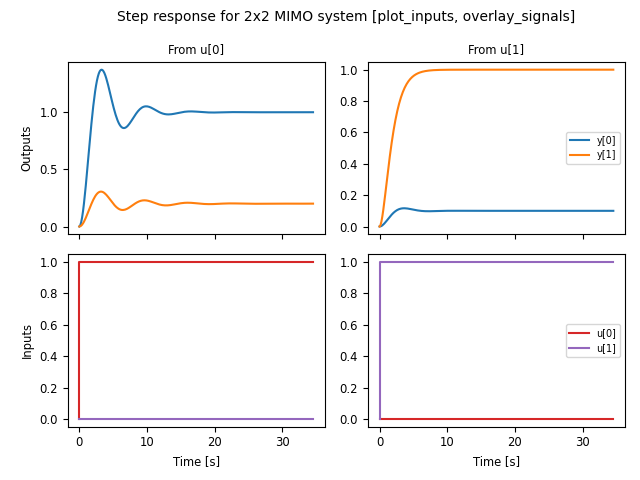Input/output response plots created with either the `forced_response()` or the `input_output_response()` functions include the input signals by default. These can be plotted on separate axes, but also “overlaid” on the output axes (useful when the input and output signals are being compared to each other). The following plot shows the use of plot_inputs=’overlay’ as well as the ability to reposition the legends using the legend_map keyword:

```timepts = np.linspace(0, 10, 100)
U = np.vstack([np.sin(timepts), np.cos(2*timepts)])
ct.input_output_response(sys_mimo, timepts, U).plot(
plot_inputs='overlay',
legend_map=np.array([['lower right'], ['lower right']]),
title="I/O response for 2x2 MIMO system " +
"[plot_inputs='overlay', legend_map]")
```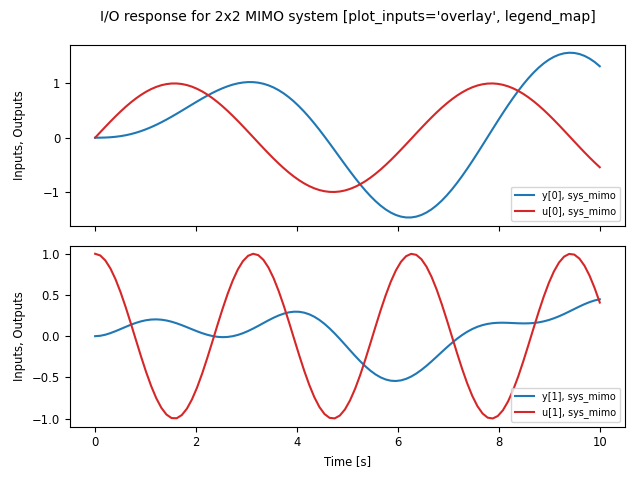Another option that is available is to use the transpose keyword so that instead of plotting the outputs on the top and inputs on the bottom, the inputs are plotted on the left and outputs on the right, as shown in the following figure:

```U1 = np.vstack([np.sin(timepts), np.cos(2*timepts)])
resp1 = ct.input_output_response(sys_mimo, timepts, U1)

U2 = np.vstack([np.cos(2*timepts), np.sin(timepts)])
resp2 = ct.input_output_response(sys_mimo, timepts, U2)

ct.combine_time_responses(
[resp1, resp2], trace_labels=["Scenario #1", "Scenario #2"]).plot(
transpose=True,
title="I/O responses for 2x2 MIMO system, multiple traces "
"[transpose]")
```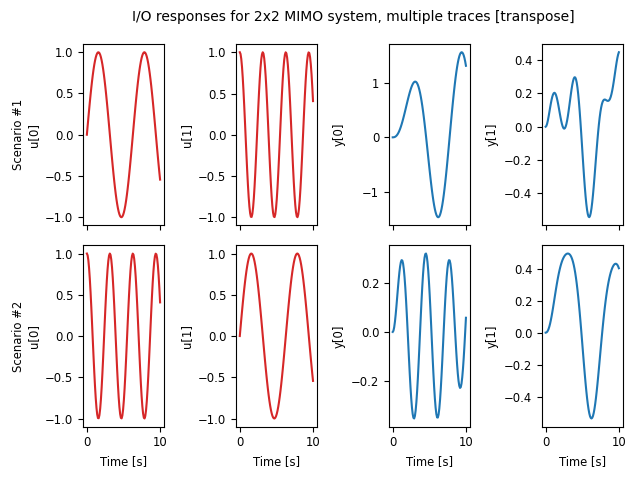This figure also illustrates the ability to create “multi-trace” plots using the `combine_time_responses()` function. The line properties that are used when combining signals and traces are set by the input_props, output_props and trace_props parameters for `time_response_plot()`.

Additional customization is possible using the input_props, output_props, and trace_props keywords to set complementary line colors and styles for various signals and traces:

```out = ct.step_response(sys_mimo).plot(
plot_inputs='overlay', overlay_signals=True, overlay_traces=True,
output_props=[{'color': c} for c in ['blue', 'orange']],
input_props=[{'color': c} for c in ['red', 'green']],
trace_props=[{'linestyle': s} for s in ['-', '--']])
```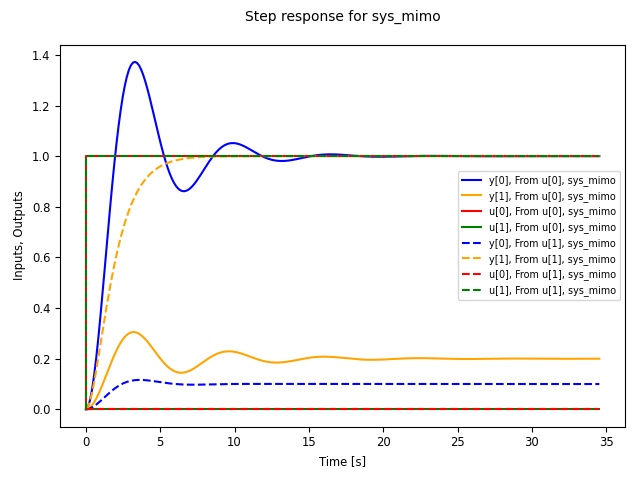## Frequency response data

Linear time invariant (LTI) systems can be analyzed in terms of their frequency response and python-control provides a variety of tools for carrying out frequency response analysis. The most basic of these is the `frequency_response()` function, which will compute the frequency response for one or more linear systems:

```sys1 = ct.tf(, [1, 2, 1], name='sys1')
sys2 = ct.tf([1, 0.2], [1, 1, 3, 1, 1], name='sys2')
response = ct.frequency_response([sys1, sys2])
```

A Bode plot provide a graphical view of the response an LTI system and can be generated using the `bode_plot()` function:

```ct.bode_plot(response, initial_phase=0)
```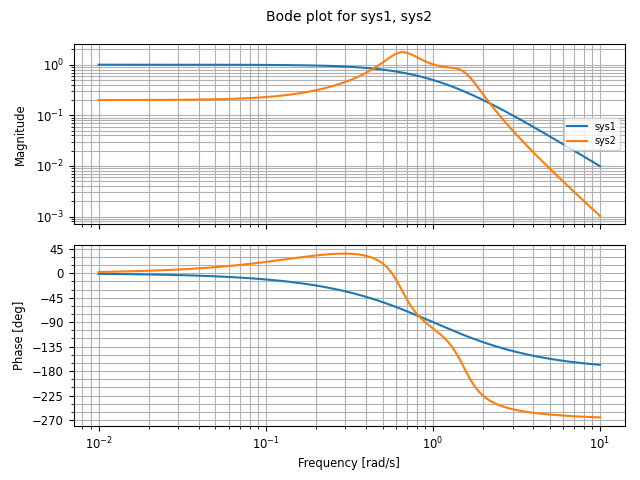Computing the response for multiple systems at the same time yields a common frequency range that covers the features of all listed systems.

Bode plots can also be created directly using the `plot()` method:

```sys_mimo = ct.tf(
[[, [0.1]], [[0.2], ]],
[[[1, 0.6, 1], [1, 1, 1]], [[1, 0.4, 1], [1, 2, 1]]], name="sys_mimo")
ct.frequency_response(sys_mimo).plot()
```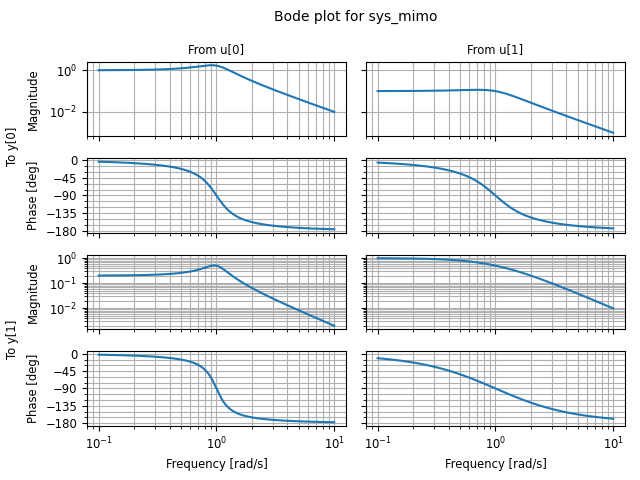A variety of options are available for customizing Bode plots, for example allowing the display of the phase to be turned off or overlaying the inputs or outputs:

```ct.frequency_response(sys_mimo).plot(
plot_phase=False, overlay_inputs=True, overlay_outputs=True)
```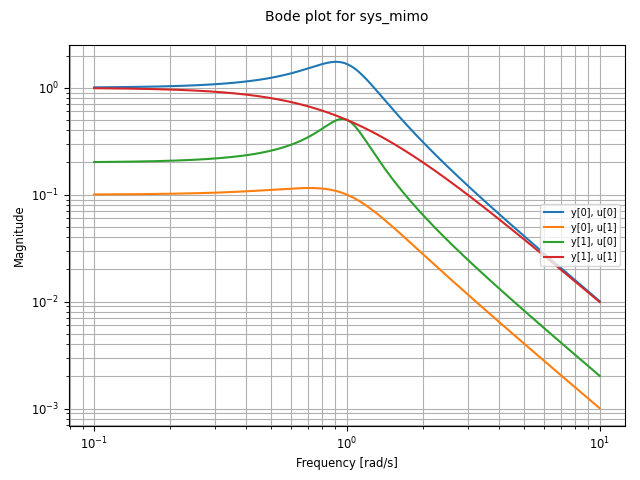The `singular_values_response()` function can be used to generate Bode plots that show the singular values of a transfer function:

```ct.singular_values_response(sys_mimo).plot()
```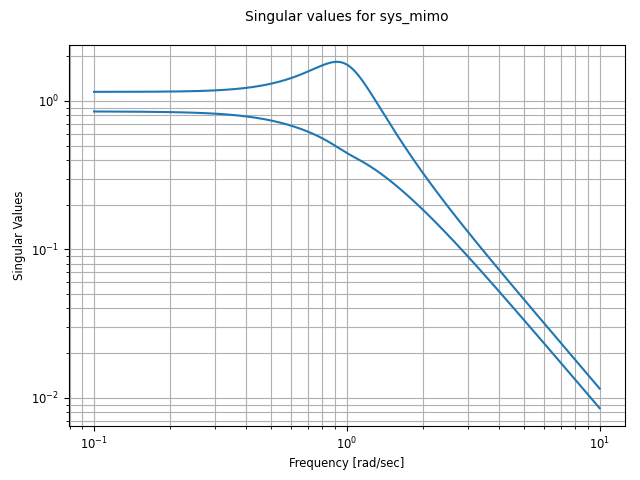Different types of plots can also be specified for a given frequency response. For example, to plot the frequency response using a a Nichols plot, use plot_type=’nichols’:

```response.plot(plot_type='nichols')
```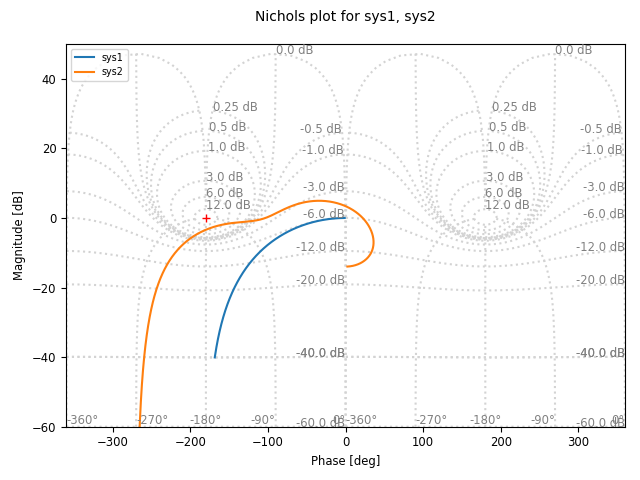Another response function that can be used to generate Bode plots is the `gangof4()` function, which computes the four primary sensitivity functions for a feedback control system in standard form:

```proc = ct.tf(, [1, 1, 1], name="process")
ctrl = ct.tf(, [1, 5], name="control")
response = rect.gangof4_response(proc, ctrl)
ct.bode_plot(response)      # or response.plot()
```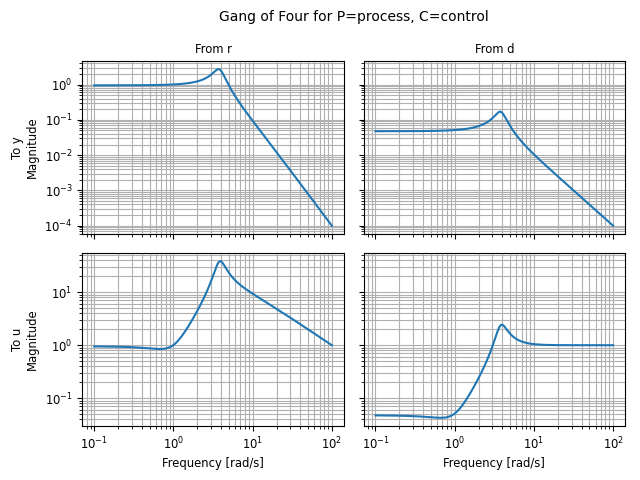## Response and plotting functions

### Response functions

Response functions take a system or list of systems and return a response object that can be used to retrieve information about the system (e.g., the number of encirclements for a Nyquist plot) as well as plotting (via the plot method).

 `describing_function_response`(H, F, A[, ...]) Compute the describing function response of a system. `frequency_response`(sysdata[, omega, ...]) Frequency response of an LTI system at multiple angular frequencies. `forced_response`(sys[, T, U, X0, transpose, ...]) Compute the output of a linear system given the input. `gangof4_response`(P, C[, omega, Hz]) Compute the response of the "Gang of 4" transfer functions for a system. `impulse_response`(sys[, T, input, output, ...]) Compute the impulse response for a linear system. `initial_response`(sys[, T, X0, output, ...]) Compute the initial condition response for a linear system. `input_output_response`(sys, T[, U, X0, ...]) Compute the output response of a system to a given input. `nyquist_response`(sysdata[, omega, plot, ...]) Nyquist response for a system. `singular_values_response`(sysdata[, omega, ...]) Singular value response for a system. `step_response`(sys[, T, X0, input, output, ...]) Compute the step response for a linear system.

### Plotting functions

 `bode_plot`(data[, omega, ax, omega_limits, ...]) Bode plot for a system. `describing_function_plot`(data, *args, **kwargs) Plot a Nyquist plot with a describing function for a nonlinear system. `nichols_plot`(data[, omega, grid, title, ...]) Nichols plot for a system. `singular_values_plot`(data[, omega, plot, ...]) Plot the singular values for a system. `time_response_plot`(data, *fmt[, ax, ...]) Plot the time response of an input/output system.

### Utility functions

These additional functions can be used to manipulate response data or returned values from plotting routines.

 `combine_time_responses`(response_list[, ...]) Combine multiple individual time responses into a multi-trace response. `get_plot_axes`(line_array) Get a list of axes from an array of lines.

### Response classes

The following classes are used in generating response data.

 `DescribingFunctionResponse`(response, N_vals, ...) Results of describing function analysis. `FrequencyResponseData`(d, w[, smooth]) A class for models defined by frequency response data (FRD). `NyquistResponseData`(count, contour, response, dt) Nyquist response data object. `TimeResponseData`(time, outputs[, states, ...]) A class for returning time responses.